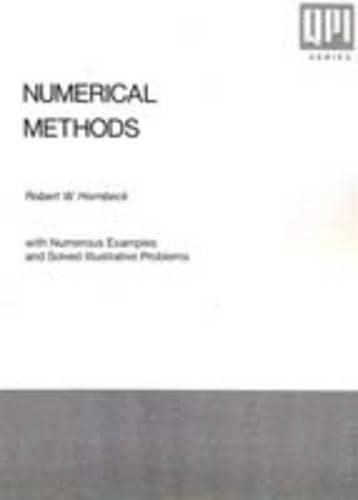# Numerical Methods by Robert W. Hornbeck

Out of Stock
\$222.99
inc. GST
N/A
Only 0 left

## Numerical Methods Summary

### Numerical Methods by Robert W. Hornbeck

Using a "learn by example" approach, this exploration of the fundamental tools of numerical methods covers both modern and older, well-established techniques that are well-suited to the digital-computer solution of problems in many areas of science and engineering.

### Why buy from World of BooksOur excellent value books literally don't cost the earthFree delivery in AustraliaEvery used book bought is one saved from landfill

1. Introductory Topics.

2. The Taylor Series.

3. The Finite Difference Calculus.

4. Interpolation and Extrapolation.

5. Roots of Equations.

6. The Solution of Simultaneous Linear Algebraic Equations and Matrix Inversion.

7. Least-Squares Curve Fitting and Functional Approximation.

8. Numerical Integration.

9. The Numerical Solution of Ordinary Differential Equations.

10. Matrix Eigenvalue Problems.

11. Introduction to Partial Differential Equations.

Appendix.

References.

Index.

GOR010177606
Numerical Methods by Robert W. Hornbeck
Robert W. Hornbeck